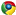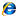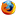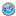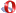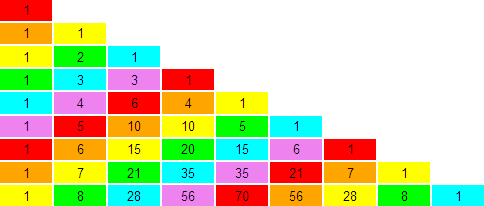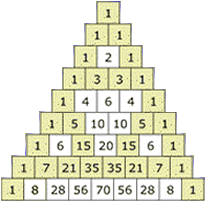The rows of Pascal's triangle are conventionally enumerated starting with row n = 0 at the top. The entries in each row are numbered from the left beginning with k = 0 and are usually staggered relative to the numbers in the adjacent rows. A simple construction of the triangle proceeds in the following manner. On row 0, write only the number 1. Then, to construct the elements of following rows, add the number directly above and to the left with the number directly above and to the right to find the new value. If either the number to the right or left is not present, substitute a zero in its place. For example, the first number in the first row is 0 + 1 = 1, whereas the numbers 1 and 3 in the third row are added to produce the number 4 in the fourth row.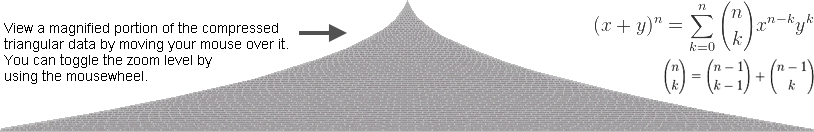On-line Query Analyser for MS-SQL ServerPlease enter the 8 digit Captcha code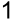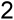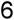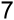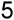-- Toggle between, 'Actual data' and 'Sierpinski Pattern', by setting @SIERPINSKI_PATTERN to '0' or '1' DECLARE @SIERPINSKI_PATTERN AS BIT SET @SIERPINSKI_PATTERN = 1 IF @SIERPINSKI_PATTERN = 0 SELECT * FROM PASCAL_TRIANGLE ELSE SELECT * FROM PASCAL_SIERPINSKI_TRIANGLE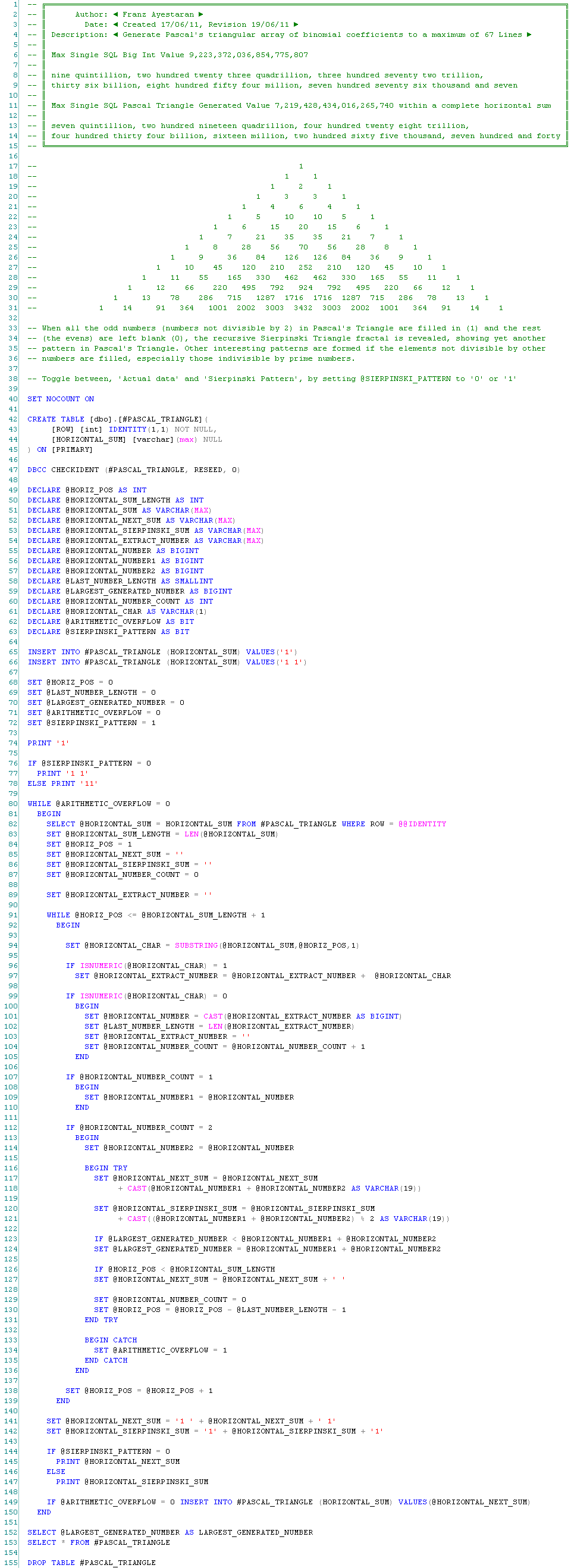Website Designed and Developed by Franz Ayestaran ©2021, Hosted | Powered by Zonk Technology
Cross-browser compatible website